Name:    Quiz 5.4-5.6

Multiple Choice
Identify the choice that best completes the statement or answers the question.

Use the Cross Products Property to solve the proportion.

1.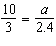a. a = 21 c. a = 8.8 b. a = 0.7 d. a = 8

2.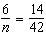a. n = 14 c. n = 18 b. n = 234 d. n = 2

3.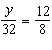a. y = 46 c. y = 44 b. y = 45 d. y = 48

4.

There are 16 fluid ounces of juice in 2 bottles of your favorite juice. How many fluid ounces are there in 10 bottles?
 a. 96 fluid ounces c. 80 fluid ounces b. 72 fluid ounces d. 160 fluid ounces

Find the slope of the line.

5.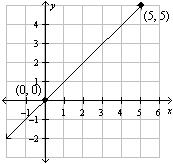a. 1 c. 5 b. 10 d.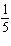6.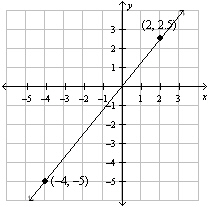a.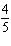c.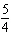b.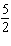d.1.

You are looking at two graphs. One shows the amount of money y that Max makes in x hours. The other graph shows the same thing for Elle. The graph for Elle is steeper. What does this tell you?

Graph the data.  Then find and interpret the slope of the line through the points.

2.

 Seconds, x 3 5 7 9 Revolutions, y 87 145 203 261

3.

 Work Days, x 5 10 15 20 Hours Worked, y 52.5 105 157.5 210

Tell whether x and y show direct variation.  Explain your reasoning. If so, find k.

4.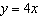5.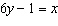6.

You rent a scooter for two days while you are on vacation. Tell whether x and y show direct variation. If so, write an equation of direct variation.

 Miles, x 50 30 Cost, y \$30 \$24